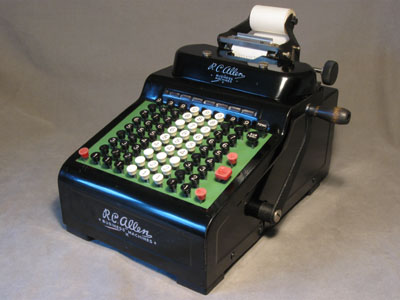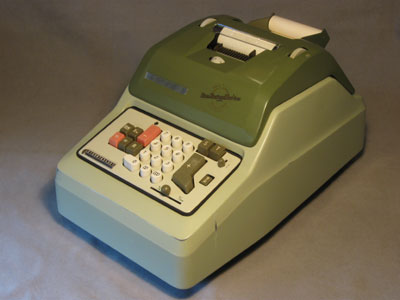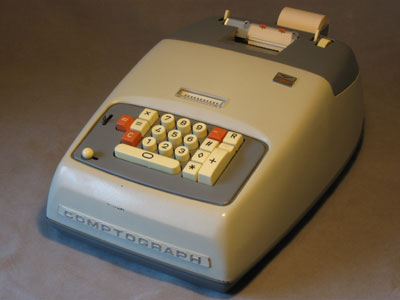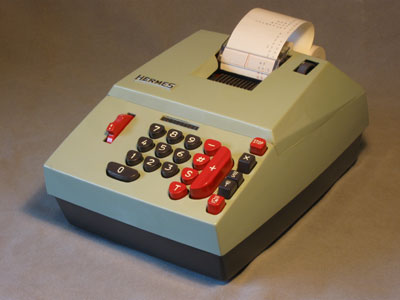MechCalc Mechanical Calculators

Manufacturer - Model Year Functions Drive Keypad Digits Display Summary R.C. Allen - 805 1930s ? Add, subtract, accumulate Crank Full 8/8 Dial, paper tape Lagomarsino - 8361 1950s ? Add, subtract, accumulate, multiply Electric 10-key 13 Paper tape Olivetti - Multisumma 22 1950s ? Add, subtract, accumulate, multiply Electric 10-key 12 Paper tape Victor - Comptograph 1960s ? Add, subtract, accumulate, multiply Electric 10-key 12 Paper tape Friden - SBT-10 1964 (introduced 1959) Add, subtract, accumulate, multiply, divide Electric Full 10/20 Dial Hermes - 309-10 1969 Add, subtract, accumulate, multiply Electric 10-key 10/11 Paper tape

A small collection of mechanical calculators, the forgotten and unloved predecessors to modern-day electronic computing equipment.

Unloved? These were the machines that gave rise to phrases like "cranking out the numbers", an expression of the tedium of working through calculations or spending one's days summing accounts with them. Even today, they don't seem to attract the same degree of interest or appeal as the space-agey electronic calculators.

Gallery
(by increasing year)R.C. Allen 805
1930s ?

 Functions: Add, subtract, accumulate Drive: Crank Keypad: Full Digits: 8/8 Display: Dial, paper tape More Photos: Yes Status: Fully functional except for problem with depressing total keys after carry (see repair 2013 Feb 03).Lagomarsino 8361
1950s ?

 Functions: Add, subtract, accumulate, multiply Drive: Electric Keypad: 10-key Digits: 13 Display: Paper tape Status: Jammed.Olivetti Multisumma 22
1950s ?

 Functions: Add, subtract, accumulate, multiply Drive: Electric Keypad: 10-key Digits: 12 Display: Paper tape Status: Addition functional.Victor Comptograph
1960s ?

 Functions: Add, subtract, accumulate, multiply Drive: Electric Keypad: 10-key Digits: 12 Display: Paper tape Status: Needs drive belt.Friden SBT-10
Key-set Electromechanical Calculator
1964 (introduced 1959)

 Functions: Add, subtract, accumulate, multiply, divide Drive: Electric Keypad: Full Digits: 10/20 Display: Dial More Photos: Yes Status: Jammed.Hermes 309-10